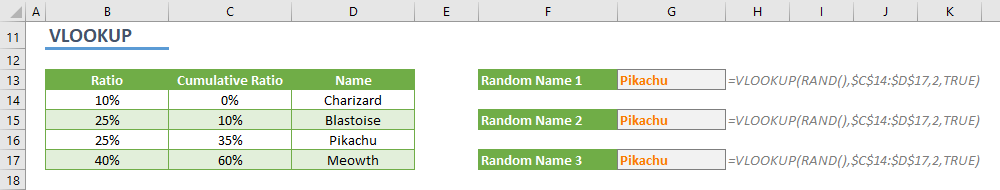Let’s say you need to populate items randomly. You can use Excel’s RANDBETWEEN function to select a random item from a list. What if the distribution of the items is not uniform? In this article, we are going to show you how to select a random item by distribution in Excel.

Although Excel doesn’t have a built-in feature or function to return a value from a list, it has RAND and RANDBETWEEN functions to return a randomly generated number. In this guide, we will use RAND function to generate a random number as well as INDEX, MATCH and VLOOKUP functions. The latter functions will help us to locate the randomly selected item.

This guide will show you how to select a random item by distribution in two ways:

1. Using INDEX and MATCH functions
2. Using VLOOKUP function

You can decide either one based on your data structure. Let’s investigate both.

## Sample data

Assume that you have a list of items with distribution ratios as below.RAND function returns a random generated number between 0 and 1. We can use the generated number to locate the corresponding item. First, define the slices corresponding to each item. We can create these slices with cumulative distributions.

Make sure the total of distribution ratio is equal to 100% and start cumulative values starting from 0. For example, if the first item’s probability ratio is 10% the first item should be 0 and the next one 10%.As a result, we need to set formulas when RAND function returns a number between 0 and 10%, it should indicate the first item. MATCH and VLOOKUP functions can be used to handle this. They can make approximate searches to locate the closest value in the list. For example, if the search value is 7.5%, approximate search assume it is 0 in our list of 0%, 10%, 35% and 60%.

Let’s see how you can combine RAND with other functions to select a random item by distribution in Excel.

## INDEX-MATCH

The first approach is using INDEX-MATCH combination which can locate and retrieve a value in a data set. The roles are clear:

• RAND returns a random number
• MATCH seeks that number in the cumulative values approximately
• INDEX return the value by using position number from MATCH function

The most important thing here is using 1 for match_type argument of MATCH function. The 1 number indicates to find the largest value that is less than or equal to the lookup value.

=INDEX(<list of values>,MATCH(RAND(),<list of cumulative ratios>,1))To learn more about using INDEX and MATCH functions together, you can check our guide: INDEX & MATCH: A Better Way to Look Up Data

## VLOOKUP

VLOOKUP is another and probably more popular way to parse values from a table. However, your data should be a table and the value should be searched in the first column. Thus, if you can or if you have to place cumulative ratios on the left side of your values, you can use VLOOKUP as well.

Like MATCH function, you have to indicate search type for VLOOKUP too. Either enter 1 or TRUE to use approximate search mode.

=VLOOKUP(RAND(),<table of values including cumulative ratios>,2,TRUE)